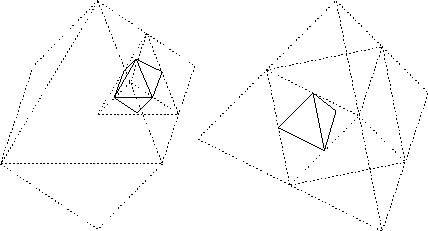Next:4.8 ExamplesUp:4. AMIGOSPrevious:4.6.2 Time Discretization

# 4.7 Grid & Element Manager

For discretization purposes one of the most important features of a simulation tool is the mesh management and grid adaptation.

To offer a wide range of possibilities AMIGOS is equipped with a powerful adaptive grid algorithm that can handle various different grid elements in all dimensions (Fig. 4.1). The Grid & Element Manager automatically detects the types of elements defined on a given mesh as well as their dimensions. The implemented algorithm supports a mixed-element decomposition method for local refinement of fully unstructured grids [Lei95][Lei97]. The method guarantees to prevent the grid quality using a hierarchical adaptation under the condition that elements of the same shape are of the same quality (Fig. 4.13). Thus the shape of elements can be preserved even in case of local anisotropies of the initial grid.

Figure 4.13: Hierarchical element decomposition for triangles, rectangles, octahedrons and tetrahedronsBecause it is hardly possible to find a general rule for grid adaptation independent from the physical problem the Analytical Model Interface allows the user to specify a grid criterion for each new developed model as an analytical expression (even tensor fields for anisotropic fittings are possible). AMIGOS uses this expression to calculate an epsilon criterion to start its adaptive hierarchical grid algorithm. Thus the local discretization can be optimized for a given physical problem.

Before starting Newton iterations over the complete PDE systems AMIGOS evaluates the grid criterion given in any defined analytical model and adapts the grid in a manner so that a maximum error of a given epsilon criterion is achieved. Afterwards it starts calculating the first Newton iteration and if necessary it readapts the mesh accounting to the changes of the grid criterions per mesh. This scheme continues until the complete system has converged.

To define a grid-criterion for a model an additional line has to be added to the model

```MODEL <modelname>=[...];
{
residuum = [...];
jacobian = [[...]];

gridcrit = <any analytical expression>
}```Next:4.8 ExamplesUp:4. AMIGOSPrevious:4.6.2 Time Discretization